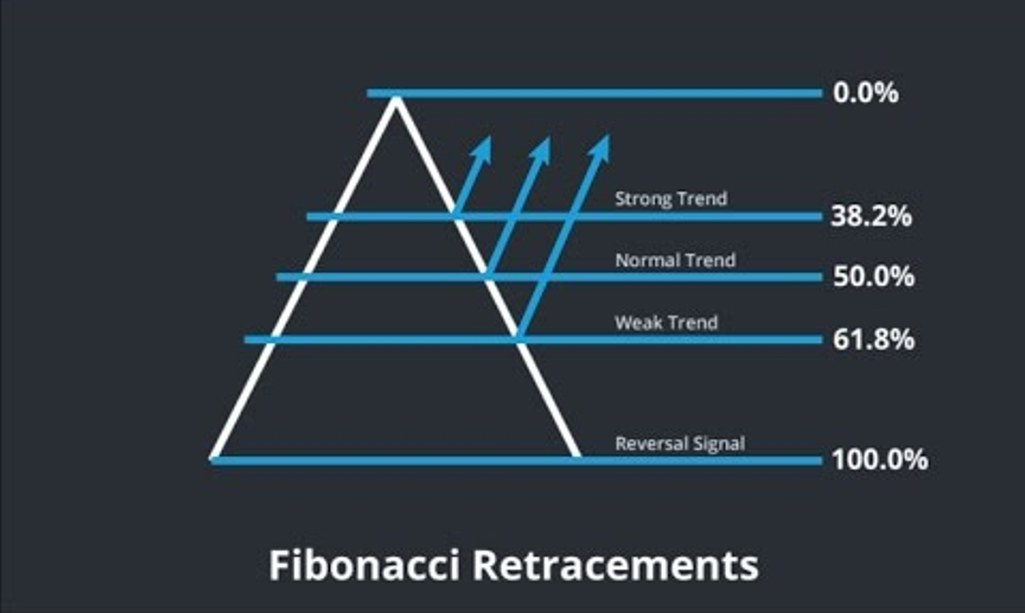# Fibonacci: Retracement

No comment 960 views
5 / 5 ( 1votes )

Fibonacci Retracement is an indicator or tool used to determine possible support and resistance levels. Like Fibonacci fans, the Fibonacci Retracements level is based on a mathematical relationship, expressed as the ratio, between the numbers in the Fibonacci sequence.Fibonacci: Retracement

The series of Fibonacci sum numbers is just the sum of the two preceding numbers beginning with 0 and 1, and continues unlimitedly. Some of the first numbers in the series are as follows: 0, 1, 1, 2, 3, 5, 8, 13, 21, 34, 55, 89, 144, Fibonacci Retracements are based on a common mathematical relationship or ratio between these numbers.

The Fibonacci retracement key level is found by performing various mathematical operations on numbers in the Fibonacci summation series, and based on the results of those operations.

The first ratio of 61.8%, which is the opposite of the “golden ratio” or “golden mean” (ie the opposite of 1.61%), is found by dividing the number in sequence by the number following it. For example: 8 ÷ 13 = 0.6153, 34 ÷ 55 = 0.6182 and 55 ÷ 89 = 0.6179.
The ratio of 38.2% is found by dividing the numbers in a sequence with numbers appearing two places to the right. For example: 8 ÷ 21 = 0.3809, 21 ÷ 55 = 0.3818 and 55 ÷ 144 = 0.3819. It is also found by subtracting 0.618 from 1 (1 – 0.618 = 0.328) and by finding the square of 0.618 (0.618 x 0.618 = 0.382).
The 23.6% ratio is found by dividing the numbers in sequence with the number that appears three places to the right. For example: 8 ÷ 34 = 0.2352, 21 ÷ 89 = 0.2359 and 34 ÷ 144 = 0.2361. It is also found by subtracting 0.382 from 0.618 (0.618 – 0.382 = 0.236) and by multiplying the ratio of 0.382 x 0.618 (0.382 x 0.618 = 0.236).
The ratio of 78.6% was found by finding the square root of 61.8%. Some traders prefer to use the 76.4% ratio found by subtracting 0.236 from 1.00 (1.00 – 0.236 = 0.764).
In addition to these four ratios, the 0% and 100% retracement levels are also used to mark the beginning of the full retracement and retracement of the previous movement, and the 50% retracement level is used to mark the midpoint between high swing and low wave swings. The 50.0% level is not the Fibonacci ratio, per se, but it is the point of attention in the retracement-fixed graph over time.
Fibonacci retracement is based on the idea that the market will retrace the predicted part of the previous price movement before the price action continues towards a larger trend. This is based on the fact that financial markets are not moving in a straight line trend. Instead the trend is interrupted by retracements that tend not to exceed previous waves.

When using Fibonacci retracements, a technical analyst waits for the market to reverse and then divides the movement of the previous price trend, or wave, with the Fibonacci retracement ratio, starting from the previous highs to the lowest in the uptrend and the reverse direction in downtrend. The horizontal line is then pulled at this level and used as a possible support level if the bigger trend is the uptrend, or maybe the resistance level if the bigger trend is the descending trend. This becomes a place where traders can buy in a larger uptrend or sell in a bigger downtrend. The most significant level is usually the 61.8% level and the 38.2% level. While the level of 23.6% and the level of 78.6% (or 76.4%) is not significant.Forex Signal 30 is the best forex system since 2009 and has been used by thousands of traders from around the world to generate profit in forex trading. This system is created by our team of Brilliant Forex Signal Team, this system is made as simple as possible for beginner and professional traders.